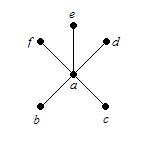# End vertex

In graph theory , a vertex with degree $1$ is called an end vertex (plural end vertices ). In the figure below, vertices $b,c,d,e$ and $f$ are end vertices.